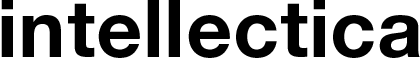# The continuum and mathematical intuitions. Problems related to mathematical intuition in Gödel's philosophy

Cassou-Noguès Pierre
Language of the article : French
DOI: 10.3406/intel.2009.1732
Product variations:

### Paper format

In 1938, Gödel announced his proof for the relative consistency of the continuum hypothesis with regards to the usual axioms of set theory. Until 1943, he devotes most of his research to an attempt to prove its independence. He will up to the end of his career continue to think, as a philosopher, about the problem of the continuum. We first distinguish different modes of intuition that appear in Gödel's writings in relation to the continuum. We then discuss the metaphysical problems that these intuitions raise. What is, for example, the role of time in mathematical intuition? If there is an abstract intuition, does it use a specific organ? If so, how should one conceive the influence of a mathematical object on a bodily organ? If mathematics has a universal value and if it requires different kinds of intuition, should we attribute these intuitions to all beings, such as God, capable of mathematics? Thus the analysis of Gödel's remarks on mathematical intuition introduces us some of the questions of his metaphysics. We essentially rely on Gödel's archives at Princeton University Library.

Pour citer cet article :

Cassou-Noguès Pierre (2009/1). The continuum and mathematical intuitions. Problems related to mathematical intuition in Gödel's philosophy. In De Glas Michel (Eds), The Mathematical Continuum. New Conceptions, New Challenges, Intellectica, 51, (pp.33-58), DOI: 10.3406/intel.2009.1732.